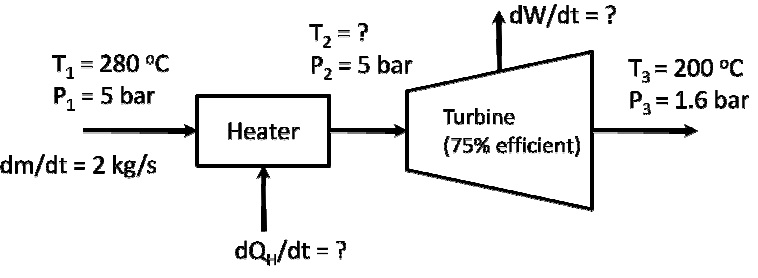Problem on entropy

A heater (heat source temperature = 527 K) and turbine are connected in series as shown below:a. Calculate the entropy generation if the process stream is steam.

b. Calculate the entropy generation if the process stream is air. You can treat air as an ideal. Calculate the entropy generation if the process stream is air. You can treat air as an ideal  Calculate the entropy generation if the process stream is air. You can treat air as an ideal gas with Cp = 27 J/mol/K and molecular weight of 29 g/mol.

#### Related Questions in Chemical Engineering

• ##### Q :Problem on heat of sublimation Using

Using the vapor pressure data provided below, estimate

i) the heat of sublimation of ice,

ii) the heat of vaporization of water,

iii) the heat of fusion of ice and compare your estimate with the published value of 6010 J/moL

iv) the triple point of wate

• ##### Q :Chemical flow I) Sulphur dioxide (SO2)

I) Sulphur dioxide (SO2) with a volumetric flow rate 5000cm3/s at 1 bar and 1000C is mixed with a second SO2 stream flowing at 2500cm3/s at 2 bar and 200C. The process occurs at steady state. You may assume ideal gas behaviour. For SO2 take the heat capacity at constant pressure to be CP/R = 3.267+5

• ##### Q :Problem on weight fraction A gas

A gas contains 350 ppm of H2S in CO2 at 72°F and 1.53 atm pressure. If the gas is liquified, what is the weight fraction H2S?

• ##### Q :Bsc what is the latent heat of

what is the latent heat of vaporization for hexane

• ##### Q :Production of ethanol Ethanol (1)

Ethanol

(1) Describe the production of ethanol in Scottish Whisky beverages. Be sure to include a discussion on dangers of impurities

(2) Explain the impact of ethanol on the human body

(3) List th

• ##### Q :How to balance Chemical Reaction

Consider a chemical reaction:

a CH3-CH=CH2 + b NH3 + c O2d CH2=CH-C≡N + e H2O

1.  &

• ##### Q :Problem on steam flow in a pipe A

A stream of steam at 15 bar and 300 oC is used to produce work using a steam turbine.

a. Before the turbine, steam flows in a pipe (4 cm in diameter) at a mass flow rate of 3 g/s. Calculate the mean velocity in the pipe

• ##### Q :Problem on laboratory solution You are

You are asked to make up a laboratory solution of 0.10 molar H2SO4 from concentrated (96%) H2SO4 at 56°F. The barometric pressure reads 750 mmHg. You look up the specific gravity of 96% H2SO4 and find it is listed at 1.858. Calculate:

(a) th

• ##### Q :Mass transfer adsorption how to

how to calculate amount of ammonia adsorbed per kg of adsobent in ammonia calcium chloride adsorption process?

• ##### Q :Thermodynamics Please can you look into

Please can you look into this assignment and let me know if its solve able.